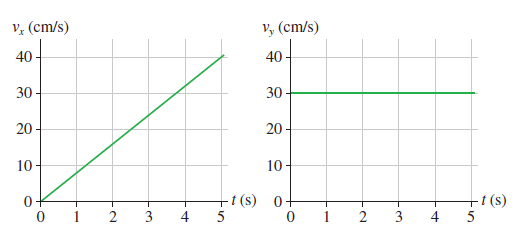# Problem: A rocket-powered hockey puck moves on a horizontal frictionless table. The figure below shows the graphs of vx and vy, the x- and y-components of the puck’s velocity. The puck starts at the origin.Part A. In which direction is the puck moving at t = 3 s? Give your answer as an angle from the x-axis. Express your answer using two significant figures.Part B. How far from the origin is the puck at 4 s? Express your answer to two significant figures and include the appropriate units.

###### FREE Expert Solution

This is a2D motion problem. We'll determine the values of velocities vx, vy,  displacements x, y, and their magnitudes.

Vector magnitude (Pythagoras theorem):

v = sqrt(vx2 + vy2)

Vector direction (Trigonometry)

Tanθ = (vy/vx)

83% (167 ratings)###### Problem Details

A rocket-powered hockey puck moves on a horizontal frictionless table. The figure below shows the graphs of vx and vy, the x- and y-components of the puck’s velocity. The puck starts at the origin.Part A. In which direction is the puck moving at t = 3 s? Give your answer as an angle from the x-axis. Express your answer using two significant figures.

Part B. How far from the origin is the puck at 4 s? Express your answer to two significant figures and include the appropriate units.